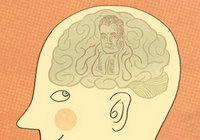## January 13, 2006

### Economist: Bayes helps explain how the mind worksThe Economist has published an excellent article, Bayes Rules, that beautifully explains not just Bayesian probability reasoning, but how our brains may actually function by using this 'technique.'

Bayes' theorem is a result in probability theory, which relates the conditional and marginal probability distributions of random variables. In some interpretations of probability, Bayes' theorem tells how to update or revise beliefs in light of new evidence.

The probability of an event A conditional on another event B is generally different from the probability of B conditional on A. However, there is a definite relationship between the two, and Bayes' theorem is the statement of that relationship. (As a formal theorem, Bayes' theorem is valid in all interpretations of probability. However, frequentist and Bayesian interpretations disagree about the kinds of variables for which the theorem holds.)

If all this means gobbedly gook to you, than I highly recommend the Economist article. It does a fairly decent job of conveying this concept in a way that releates quite well to real life decision making in light in insufficient data.
The key to successful Bayesian reasoning is not in having an extensive, unbiased sample, which is the eternal worry of frequentists, but rather in having an appropriate “prior”, as it is known to the cognoscenti. This prior is an assumption about the way the world works—in essence, a hypothesis about reality—that can be expressed as a mathematical probability distribution of the frequency with which events of a particular magnitude happen.
For example, "the participants [in the Bayesian study] were asked about things as diverse as the number of lines in a poem (given how far into the poem a single line is), the time it takes to bake a cake (given how long it has already been in the oven), and the total length of the term that would be served by an American congressman (given how long he has already been in the House of Representatives). All of these things have well-established probability distributions, and all of them, together with three other items on the list—an individual's lifespan given his current age, the run-time of a film, and the amount of time spent on hold in a telephone queuing system—were predicted accurately by the participants from lone pieces of data."

I'm reminded of AI researcher and Singularitarian Eliezer Yudkowsky who has insisted for years that this is how the brain works, and that this is the key to not just understanding our own minds, but that of artificial (super)intelligence as well. For more information, Yudkowsky has written a An Intuitive Explanation of Bayesian Reasoning.

Tags: , , , .